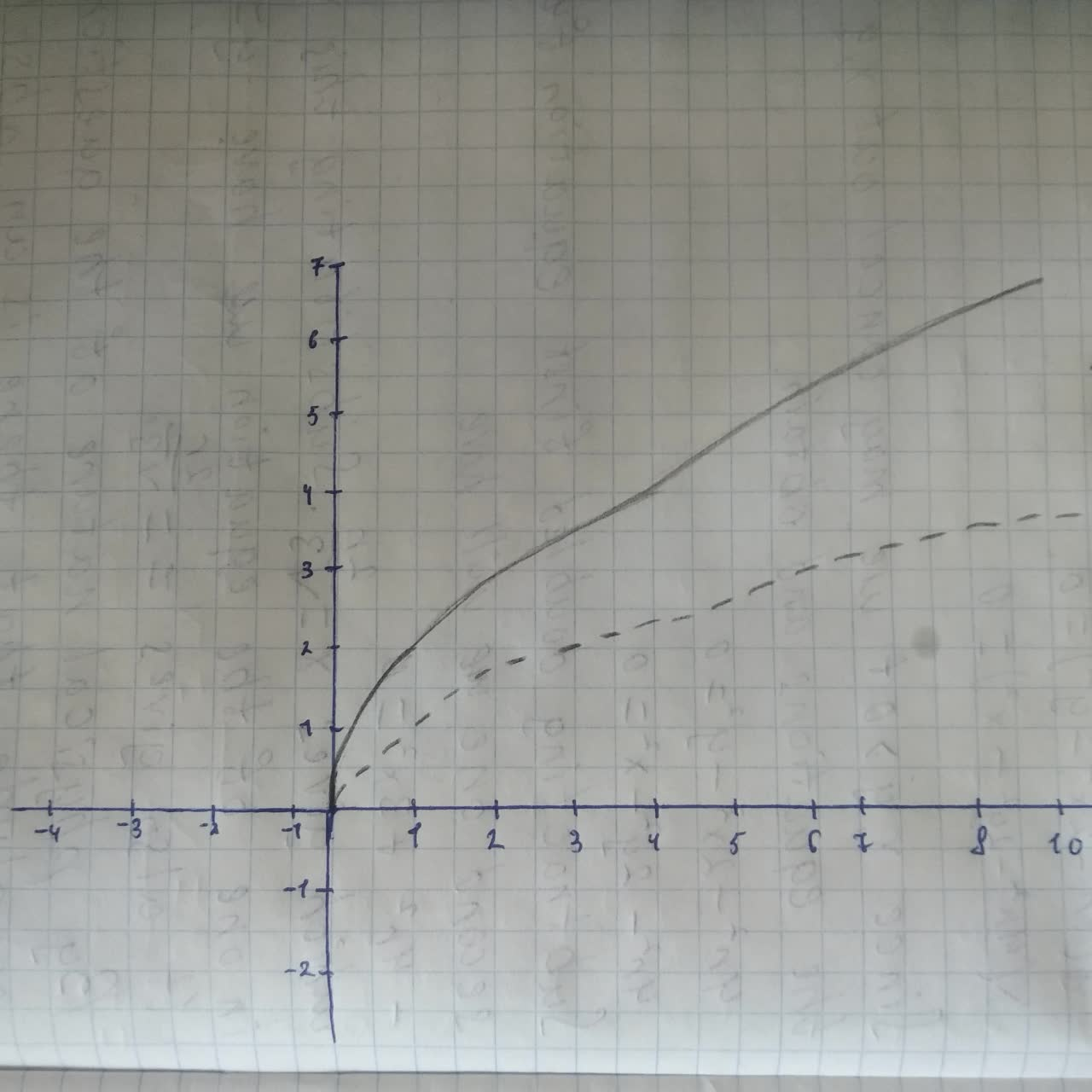# Graph the function by hand, not by plotting points, buthexacordoK 2021-08-14 Answered
Graph the function by hand, not by plotting points, but by starting with the graph of one of the standard functions, and then applying the appropriate transformations.
$y=2\sqrt{x+1}$
You can still ask an expert for help

• Questions are typically answered in as fast as 30 minutes

Solve your problem for the price of one coffee

• Math expert for every subject
• Pay only if we can solve itYusuf Keller

We will start with the graph of the standard/parent function $y=\sqrt{x}$
The graph of $y=k\cdot f\left(x\right)$ is obtained by vertically stretching the graph of $y=f\left(x\right)$ by a factor of k.
Therefore, if we vertically stretch the graph of $y=\sqrt{x}$ by a factor of 2, we will get the graph of $y=2\sqrt{x}$
In the graph below:
The blue dashed curve represents $y=\sqrt{x}$
The red solid curve represents $y=2\sqrt{x}$